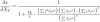# Integration step too small for ODE equationHello,

I'm using IntegrateODE to fit a binding curve in a titration experiment. The general solution for the differential equation with respect to the amount titrated is shown in the image. However, the integrator shows the following error: "integration step size has gotten too small", even when I don't have any parameters set for the error tolerance. I've also tried using different methods (\M=(1..3)) with a similar outcome.

I've been able to work around it by solving the product of the polynomials on the numerator that follows Mt and then multiply everything by Sum(betai * xj)2, but at the moment I'm limited to polynomials of degree 2 and 3 (j=2,3)

1) Does anyone have an idea on how to get around the "step size" issue so that I can leave the polynomial in its native form ?

2) I've been reading on how to implement matrices to solve this problem, but this has been a dead end so far. Does anyone know of a simple problem that uses matrices to fit partition functions of this type?

Thanks

Isaac H.iherrera wrote:

1) Does anyone have an idea on how to get around the "step size" issue so that I can leave the polynomial in its native form ?

It depends on exactly why the step size has gotten too small. Polynomials can be quite difficult to compute, since x^j can get very large or very small when X is even modestly different from 1. You may be having trouble with numerical stability, in which case the only solution is to find a more stable way to compute the polynomials, or you might have to use normalized values of x to keep them in a range around 1.

I can't tell if your system is stiff just by looking at the equation; if it is, it should have yielded to /M=3. My bet is on numerical instability.

If you can't make progress, you could send me an Igor experiment containing your code and commands to run IntegrateODE.

John Weeks
WaveMetrics, Inc.
support@wavemetrics.com
Thanks John,

I was able to obtain better results by normalizing like you mentioned. I divided two of the polynomials by x, so now I have x*(Sum(j*beta_j * x^(j-1)))^2 and Sum(j^2*beta_j * x^(j-1)) as part of my differential equation. This will allow me to implement a function for self generating polynomials in cases where j>3. I'll send you my experiment if I run into a dead end again.

My guess is that IntegrateODE doesn't handle well equations of the type dx/dy= 1/(1+g(x)/f(x)) when you can write a similar equation that looks like dx/dy = f(x)/(f(x)+g(x)).

iherrera wrote:
My guess is that IntegrateODE doesn't handle well equations of the type dx/dy= 1/(1+g(x)/f(x)) when you can write a similar equation that looks like dx/dy = f(x)/(f(x)+g(x)).

I don't think it's IntegrateODE that has the problem- it's just floating-point arithmetic on a computer. If g(x)/f(x) is very small, because of the limited resolution of digital floating-point numbers, you lose precision when you add it to 1. The alternate form may be more robust numerically.

John Weeks
WaveMetrics, Inc.
support@wavemetrics.com